Hot air balloon

Hot air balloon ascends 25 meters up for a minute after launch. Every minute ascends 75 percent of the height which climbed in the previous minute.

a) how many meters ascends six minutes after takeoff?
b) what is the overall height 10 minutes after launch?
c) how long take the balloon to gain over 110 meters high?
d) what will be the final height of the balloon?

Result

a =  5.93 m
b =  94.37 m
t =  NAN min
d =  100 m

Solution:Leave us a comment of this math problem and its solution (i.e. if it is still somewhat unclear...):Be the first to comment!Next similar math problems:

1. Balls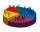We have n identical balls (numbered 1-n) is selected without replacement. Determine 1) The probability that at least one tensile strength number coincides with the number of balls? 2) Determine the mean and variance of the number of balls, which coincides.
2. Piano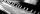If Suzan practicing 10 minutes at Monday; every other day she wants to practice 2 times as much as the previous day, how many hours and minutes will have to practice on Friday?
3. Virus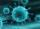We have a virus that lives one hour. Every half hour produce two child viruses. What will be the living population of the virus after 3.5 hours?
4. A perineumA perineum string is 10% shorter than its original string. The first string is 24, what is the 9th string or term?
5. Theorem proveWe want to prove the sentence: If the natural number n is divisible by six, then n is divisible by three. From what assumption we started?
6. Six termsFind the first six terms of the sequence a1 = -3, an = 2 * an-1
7. Geometric progression 2There is geometric sequence with a1=5.7 and quotient q=-2.5. Calculate a17.
8. Sum of seriesDetermine the 6-th member and the sum of a geometric series: 5-4/1+16/5-64/25+256/125-1024/625+....
9. Geometric progression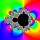Fill 4 numbers between 4 and -12500 to form geometric progression.
10. Tenth memberCalculate the tenth member of geometric sequence when given: a1=1/2 and q=2
11. Five membersWrite first 5 members geometric sequence and determine whether it is increasing or decreasing: a1 = 3 q = -2
12. Inverse matrix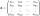Find how many times is the larger determinant is the matrix A, which equals 9 as the determinant of its inverse matrix.
13. GP membersThe geometric sequence has 10 members. The last two members are 2 and -1. Which member is -1/16?
14. Geometric sequence 4It is given geometric sequence a3 = 7 and a12 = 3. Calculate s23 (= sum of the first 23 members of the sequence).
15. The determinantThe determinant of the unit matrix equals 7. Check how many rows the A matrix contains.
16. GP - 8 itemsDetermine the first eight members of a geometric progression if a9=512, q=2
17. Geometric progression 48,4√2,4,2√2